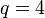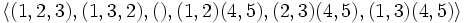# Alternating group:A5

View a complete list of particular groups (this is a very huge list!)[SHOW MORE]

## Definition

The alternating group$A_5$, also denoted$\operatorname{Alt}(5)$, and termed the alternating group of degree five, is defined in the following ways:

• It is the group of even permutations (viz., the alternating group) on five elements.
• It is the von Dyck group (sometimes termed triangle group, though the latter has a slightly different meaning) with parameters$(5,3,2)$.
• It is the icosahedral group, i.e., the group of orientation-preserving symmetries of the regular icosahedron (or equivalently, regular dodecahedron). Viewed this way, it is denoted$l$ or$532$. Further information: Classification of finite subgroups of SO(3,R), Linear representation theory of alternating group:A5
• It is the projective special linear group of degree two over the field of four elements, viz.,$PSL(2,4)$. It is also the special linear group of degree two over the field of four elements, i.e.,$SL(2,4)$. It is also the projective general linear group of degree two over the field of four elements, i.e.,$PGL(2,4)$.
• It is the projective special linear group of degree two over the field of five elements, viz.,$PSL(2,5)$.

## Arithmetic functions

Function Value Similar groups Explanation
order (number of elements, equivalently, cardinality or size of underlying set) 60 groups with same order$5!/2 = 60$.
exponent of a group 30 groups with same order and exponent of a group | groups with same exponent of a group Elements of order$2,3,5$.
derived length -- -- not a solvable group.
nilpotency class -- -- not a nilpotent group.
Frattini length 1 groups with same order and Frattini length | groups with same Frattini length Frattini-free group: intersection of maximal subgroups is trivial.
minimum size of generating set 2 groups with same order and minimum size of generating set | groups with same minimum size of generating set$(1,2,3), (1,2,3,4,5)$.
subgroup rank of a group 2 groups with same order and subgroup rank of a group | groups with same subgroup rank of a group --
max-length of a group 4 groups with same order and max-length of a group | groups with same max-length of a group --
composition length 1 groups with same order and composition length | groups with same composition length --
chief length 1 groups with same order and chief length | groups with same chief length --

### Arithmetic functions of a counting nature

Function Value Explanation
number of subgroups 59 --
number of conjugacy classes 5 As$PSL(2,q)$,$q = 5$:$(q + 5)/2 = (5 + 5)/2 = 5$, as$PGL(2,q)$,$q = 4$ a prime power:$q + 1 = 4 + 1 = 5$.
number of conjugacy classes of subgroups 9

## Group properties

Property Satisfied Explanation Comment
Abelian group No$(1,2,3)$,$(1,2,3,4,5)$ don't commute$A_n$ is non-abelian,$n \ge 4$.
Nilpotent group No Centerless: The center is trivial$A_n$ is non-nilpotent,$n \ge 4$.
Metacyclic group No Simple and non-abelian$A_n$ is not metacyclic,$n \ge 4$.
Supersolvable group No Simple and non-abelian$A_n$ is not supersolvable,$n \ge 4$.
Solvable group No$A_n$ is not solvable,$n \ge 5$.
Simple group Yes Smallest simple non-abelian group
T-group Yes Simple and non-abelian
Rational-representation group No
Rational group No
Ambivalent group Yes Also see classification of ambivalent alternating groups
Complete group No Conjugation by odd permutations of$S_5$ gives outer automorphisms

## Endomorphisms

### Automorphisms

The automorphism group of$A_5$ is the symmetric group on five letters$S_5$, with$A_5$ embedded in it as inner automorphisms.

Concretely, we can think of$A_5$ as embedded in$S_5$, and$S_5$ acting on$A_5$ by conjugation. The automorphisms obtained this way are all the automorphisms of$A_5$.

### Other endomorphisms

Since$A_5$ is a finite simple group, it is a group in which every endomorphism is trivial or an automorphism. In particular, the endomorphisms of$A_5$ are: the trivial map, and the automorphisms described above.

## Elements

Further information: element structure of alternating group:A5

### Upto conjugacy

Further information: Splitting criterion for conjugacy classes in the alternating group

There are the following conjugacy classes. These correspond to the ways of partitioning$5$ as sums of numbers where the number of even numbers is even; the partitions where all the parts are odd and distinct give rise to two conjugacy classes:

1.$5 = 1 + 1 + 1 + 1 + 1$, five fixed points: The identity element. (1)
2.$5 = 2 + 2 + 1$, two transpositions and one fixed point: The conjugacy class of double transpositions, such as$(1,2)(3,4)$. (15)
3.$5 = 3 + 1 + 1$, one 3-cycle and two fixed points: The conjugacy class of 3-cycles. (20)
4.$5 = 5$: The conjugacy class of 5-cycles conjugate to$(1,2,3,4,5)$. (12)
5.$5= 5$: The conjugacy class of 5-cycles conjugate to$(1,3,5,2,4)$. (12)

### Upto automorphism

Under outer automorphisms, the fourth and fifth conjugacy classes get merged. Thus, the classes under automorphism are of size$1,15,20,24$.

## Subgroups

Further information: Subgroup structure of alternating group:A5

Here is a complete list of subgroups of$A_5$.

1. The trivial subgroup. (1)
2. The two-element subgroup generated by a double transposition, such as$(1,2)(3,4)$. Isomorphic to Cyclic group:Z2.(15)
3. The four-element subgroup comprising all double transpositions on four of the five elements, such as$\langle (), (1,2)(3,4), (1,3)(2,4), (1,4)(2,3) \rangle$. Isomorphic to Klein four-group.(5)
4. The three-element subgroup generated by a 3-cycle, such as$(1,2,3)$. Isomorphic to cyclic group:Z3.(10)
5. A six-element subgroup that is isomorphic to the symmetric group on three letters. This moves three elements as the symmetric group on those three elements does, while it transposes the other two elements iff it is odd. For instance,$\langle (1,2,3), (1,3,2), (), (1,2)(4,5), (2,3)(4,5), (1,3)(4,5) \rangle$. (10)
6. A twelve-element subgroup that is the alternating group on four of the five letters. (5)
7. A five-element subgroup generated by a 5-cycle. Isomorphic to cyclic group:Z5. (6)
8. A ten-element subgroup generated by a 5-cycle and a double transposition that conjugates it to its inverse. Isomorphic to dihedral group:D10. (6)
9. The whole group. (1)

## Bigger groups

### Groups having it as a subgroup

The alternating group is a subgroup of index two inside the symmetric group on five elements. It is also of index two in the full icosahedral symmetry group, which turns out not to be$S_5$, but instead the direct product of$A_5$ and the cyclic group of order two.

### Groups having it as a quotient

The alternating group is a quotient of$SL(2,5)$ by its center. Hence, it is the inner automorphism group of$SL(2,5)$.$SL(2,5)$ is also the universal central extension of the alternating group.

## GAP implementation

### Group ID

This finite group has order 60 and has ID 5 among the groups of order 60 in GAP's SmallGroup library. For context, there are 13 groups of order 60. It can thus be defined using GAP's SmallGroup function as:

SmallGroup(60,5)

For instance, we can use the following assignment in GAP to create the group and name it$G$:

gap> G := SmallGroup(60,5);

Conversely, to check whether a given group$G$ is in fact the group we want, we can use GAP's IdGroup function:

IdGroup(G) = [60,5]

or just do:

IdGroup(G)

to have GAP output the group ID, that we can then compare to what we want.

### Other descriptions

The group can be defined using the AlternatingGroup function as:

AlternatingGroup(5)